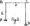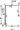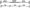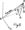# Civil Engineering Theory of Structures-1 Dec 2011

UNIT- I

(a) Define static and kinematic indeterminacy. Determine the static and kinematic indeterminacy of the following structures shown in Fig 1 (a) and(b).(b) Write Maxwell Betti’s generalized theorem. Using Maxwell- Betti’s generalized theorem, find out the vertical deflection at F due to a horizontal load of 20 kN at E towards right and an anticlockwise moment of 1.6 kN-m acting at D. It is also given that a vertical load of 20 kN acting at F in the portal frame shown in Fig. 2 produces a horizontal deflection of 1mm at E towards left and a clockwise rotation of 0.1 radians at D.1 (a) Write Moment Area Theorems.

Find the slope and deflection at a quarter span for a fixed beam loaded by point load at the centre of the span.

(b) Write and prove Maxwell’s reciprocal theorem.

UNIT-II

Analyse the frame shown in Fig.3 using slope-defiection method. Draw BMD and deflected shape.

OR

Analyze the frame shown in Fig. 3 using moment distribution method. Draw BMD and deflected shape.UNIT-III

(a) A beam AB of span L is fixed at both the ends and carries a point load W at its centre. The moment of inertia of first half portion of the beam is 21 and that of next half is I. Calculate the fixed end moments using column analogy method.

(b)   Using column analogy method determine the stiffness at A and carry over from A to B for the beam shown in Fig. 4.OR

(a) What are the analogies made in column analogy method ? Derive the generalized column flexure formula to calculate the stress f while analysing the portal frame using column analogy method having indeterminancy upto three.

(b) A continuous beam is shown in Fig. 5. Find out the support reactions using conjugate beam method.

UNIT-IV

4    A warren truss of 12 m span is built up of equilateral triangles of 4m sides. It carries equal loads at the lower joists such that the tension members are stressed to 120. N/mm2, while the compression members are stressed to 100 N/mm2. The end A is hinged and the end D is freely supported over rollers. Calculate the vertical deflection under each load, taking E = 2 x 105 N/mm2. The truss is shown in Fig-6.

(b)In the pin jointed truss shown in Fig. 7, the members AB, BC and CA have area of cross section £2a’ and members DA, DB and DC have area of cross section as £a\ Find the force in the member DA due to a load of 100 kN applied horizontally at A.UNIT-V

(a) State the assumptions made and procedure applied on Factor Method for analysing building frames with lateral loads.

(b) A pair of shear leg has lengths of each leg as 5m and the distance between their feet is 4m. The line jointing the feet of legs is 7m from the foot of the guy rope. If the length of the guy rope is 10 m, find the trust in each leg and pull in the guy rope when a load of 100 kN is suspended from the head.Fig. 8

OR

(a) Write the assumptions made in cantilever method for lateral load analysis of frames.

(b) Using Portal method, analyze the portal frame given in Fig. 9 All bays are 4 m wide Each story height is 4 m